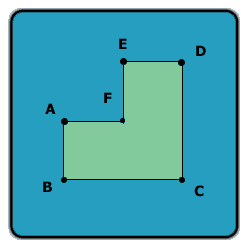All about flooble | fun stuff | Get a free chatterbox | Free JavaScript | Avatarsperplexus dot infoA different angle (Posted on 2003-10-10)Look at this shape:Assume AB = AF = FE = ED and BC = CD, and all the angles in the shape are 90 degrees.

Let A be the area of this shape (in cm^2), and P -- the perimeter of this shape (in cm).

If A - P is 60, what is the length of line AC?

 See The Solution Submitted by Lewis Rating: 1.9167 (12 votes)Comments: ( Back to comment list | You must be logged in to post comments.)is this a bit too easy?| Comment 21 of 23 |Let AB = n, then BC = 2n

Area = (2n)^2 - n^2 = 3n^2

P = 4(n) + 2 (2n) = 8n

A-P = 3n^2 - 8n = 60

3n^2 - 8n - 60 = 0

(n - 6) (3n + 10) = 0

n = 6 or - 3/10 (rejected)

AC^2 = 6(6) + (12)(12)

AC = 13.42

well

Edited on October 15, 2005, 4:19 am

Edited on October 15, 2005, 4:20 am
 Posted by Terence on 2005-10-15 04:18:32Please log in:

 Search: Search body:
Forums (5)